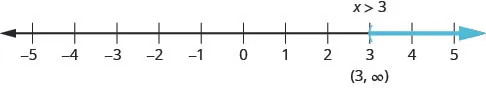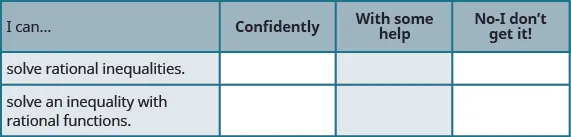Intermediate Algebra

# 7.6Solve Rational Inequalities

Intermediate Algebra7.6 Solve Rational Inequalities

### Learning Objectives

By the end of this section, you will be able to:
• Solve rational inequalities
• Solve an inequality with rational functions

### Be Prepared 7.6

Before you get started, take this readiness quiz.

1. Find the value of $x−5x−5$ when $x=6x=6$ $x=−3x=−3$ $x=5.x=5.$
If you missed this problem, review Example 1.6.
2. Solve: $8−2x<12.8−2x<12.$
If you missed this problem, review Example 2.52.
3. Write in interval notation: $−3≤x<5.−3≤x<5.$
If you missed this problem, review Example 2.49.

### Solve Rational Inequalities

We learned to solve linear inequalities after learning to solve linear equations. The techniques were very much the same with one major exception. When we multiplied or divided by a negative number, the inequality sign reversed.

Having just learned to solve rational equations we are now ready to solve rational inequalities. A rational inequality is an inequality that contains a rational expression.

### Rational Inequality

A rational inequality is an inequality that contains a rational expression.

Inequalities such as $32x>1,2xx−3<4,2x−3x−6≥x,32x>1,2xx−3<4,2x−3x−6≥x,$ and $14−2x2≤3x14−2x2≤3x$ are rational inequalities as they each contain a rational expression.

When we solve a rational inequality, we will use many of the techniques we used solving linear inequalities. We especially must remember that when we multiply or divide by a negative number, the inequality sign must reverse.

Another difference is that we must carefully consider what value might make the rational expression undefined and so must be excluded.

When we solve an equation and the result is $x=3,x=3,$ we know there is one solution, which is 3.

When we solve an inequality and the result is $x>3,x>3,$ we know there are many solutions. We graph the result to better help show all the solutions, and we start with 3. Three becomes a critical point and then we decide whether to shade to the left or right of it. The numbers to the right of 3 are larger than 3, so we shade to the right.To solve a rational inequality, we first must write the inequality with only one quotient on the left and 0 on the right.

Next we determine the critical points to use to divide the number line into intervals. A critical point is a number which make the rational expression zero or undefined.

We then will evaluate the factors of the numerator and denominator, and find the quotient in each interval. This will identify the interval, or intervals, that contains all the solutions of the rational inequality.

We write the solution in interval notation being careful to determine whether the endpoints are included.

### Example 7.54

Solve and write the solution in interval notation: $x−1x+3≥0.x−1x+3≥0.$

### Try It 7.107

Solve and write the solution in interval notation: $x−2x+4≥0.x−2x+4≥0.$

### Try It 7.108

Solve and write the solution in interval notation: $x+2x−4≥0.x+2x−4≥0.$

We summarize the steps for easy reference.

### How To

#### Solve a rational inequality.

1. Step 1. Write the inequality as one quotient on the left and zero on the right.
2. Step 2. Determine the critical points–the points where the rational expression will be zero or undefined.
3. Step 3. Use the critical points to divide the number line into intervals.
4. Step 4. Test a value in each interval. Above the number line show the sign of each factor of the numerator and denominator in each interval. Below the number line show the sign of the quotient.
5. Step 5. Determine the intervals where the inequality is correct. Write the solution in interval notation.

The next example requires that we first get the rational inequality into the correct form.

### Example 7.55

Solve and write the solution in interval notation: $4xx−6<1.4xx−6<1.$

### Try It 7.109

Solve and write the solution in interval notation: $3xx−3<1.3xx−3<1.$

### Try It 7.110

Solve and write the solution in interval notation: $3xx−4<2.3xx−4<2.$

In the next example, the numerator is always positive, so the sign of the rational expression depends on the sign of the denominator.

### Example 7.56

Solve and write the solution in interval notation: $5x2−2x−15>0.5x2−2x−15>0.$

### Try It 7.111

Solve and write the solution in interval notation: $1x2+2x−8>0.1x2+2x−8>0.$

### Try It 7.112

Solve and write the solution in interval notation: $3x2+x−12>0.3x2+x−12>0.$

The next example requires some work to get it into the needed form.

### Example 7.57

Solve and write the solution in interval notation: $13−2x2<53x.13−2x2<53x.$

### Try It 7.113

Solve and write the solution in interval notation: $12+4x2<3x.12+4x2<3x.$

### Try It 7.114

Solve and write the solution in interval notation: $13+6x2<3x.13+6x2<3x.$

### Solve an Inequality with Rational Functions

When working with rational functions, it is sometimes useful to know when the function is greater than or less than a particular value. This leads to a rational inequality.

### Example 7.58

Given the function $R(x)=x+3x−5,R(x)=x+3x−5,$ find the values of x that make the function less than or equal to 0.

### Try It 7.115

Given the function $R(x)=x−2x+4,R(x)=x−2x+4,$ find the values of x that make the function less than or equal to 0.

### Try It 7.116

Given the function $R(x)=x+1x−4,R(x)=x+1x−4,$ find the values of x that make the function less than or equal to 0.

In economics, the function $C(x)C(x)$ is used to represent the cost of producing x units of a commodity. The average cost per unit can be found by dividing $C(x)C(x)$ by the number of items $x.x.$ Then, the average cost per unit is $c(x)=C(x)x.c(x)=C(x)x.$

### Try It 7.118

The function $C(x)=5x+900C(x)=5x+900$ represents the cost to produce $x,x,$ number of items. Find the average cost function, $c(x)c(x)$ how many items should be produced so that the average cost is less than \$20?

### Section 7.6 Exercises

#### Practice Makes Perfect

Solve Rational Inequalities

In the following exercises, solve each rational inequality and write the solution in interval notation.

339.

$x − 3 x + 4 ≥ 0 x − 3 x + 4 ≥ 0$

340.

$x + 6 x − 5 ≥ 0 x + 6 x − 5 ≥ 0$

341.

$x + 1 x − 3 ≤ 0 x + 1 x − 3 ≤ 0$

342.

$x − 4 x + 2 ≤ 0 x − 4 x + 2 ≤ 0$

343.

$x − 7 x − 1 > 0 x − 7 x − 1 > 0$

344.

$x + 8 x + 3 > 0 x + 8 x + 3 > 0$

345.

$x − 6 x + 5 < 0 x − 6 x + 5 < 0$

346.

$x + 5 x − 2 < 0 x + 5 x − 2 < 0$

347.

$3 x x − 5 < 1 3 x x − 5 < 1$

348.

$5 x x − 2 < 1 5 x x − 2 < 1$

349.

$6 x x − 6 > 2 6 x x − 6 > 2$

350.

$3 x x − 4 > 2 3 x x − 4 > 2$

351.

$2 x + 3 x − 6 ≤ 1 2 x + 3 x − 6 ≤ 1$

352.

$4 x − 1 x − 4 ≤ 1 4 x − 1 x − 4 ≤ 1$

353.

$3 x − 2 x − 4 ≥ 2 3 x − 2 x − 4 ≥ 2$

354.

$4 x − 3 x − 3 ≥ 2 4 x − 3 x − 3 ≥ 2$

355.

$1 x 2 + 7 x + 12 > 0 1 x 2 + 7 x + 12 > 0$

356.

$1 x 2 − 4 x − 12 > 0 1 x 2 − 4 x − 12 > 0$

357.

$3 x 2 − 5 x + 4 < 0 3 x 2 − 5 x + 4 < 0$

358.

$4 x 2 + 7 x + 12 < 0 4 x 2 + 7 x + 12 < 0$

359.

$2 2 x 2 + x − 15 ≥ 0 2 2 x 2 + x − 15 ≥ 0$

360.

$6 3 x 2 − 2 x − 5 ≥ 0 6 3 x 2 − 2 x − 5 ≥ 0$

361.

$−2 6 x 2 − 13 x + 6 ≤ 0 −2 6 x 2 − 13 x + 6 ≤ 0$

362.

$−1 10 x 2 + 11 x − 6 ≤ 0 −1 10 x 2 + 11 x − 6 ≤ 0$

363.

$1 2 + 12 x 2 > 5 x 1 2 + 12 x 2 > 5 x$

364.

$1 3 + 1 x 2 > 4 3 x 1 3 + 1 x 2 > 4 3 x$

365.

$1 2 − 4 x 2 ≤ 1 x 1 2 − 4 x 2 ≤ 1 x$

366.

$1 2 − 3 2 x 2 ≥ 1 x 1 2 − 3 2 x 2 ≥ 1 x$

367.

$1 x 2 − 16 < 0 1 x 2 − 16 < 0$

368.

$4 x 2 − 25 > 0 4 x 2 − 25 > 0$

369.

$4 x − 2 ≥ 3 x + 1 4 x − 2 ≥ 3 x + 1$

370.

$5 x − 1 ≤ 4 x + 2 5 x − 1 ≤ 4 x + 2$

Solve an Inequality with Rational Functions

In the following exercises, solve each rational function inequality and write the solution in interval notation.

371.

Given the function $R(x)=x−5x−2,R(x)=x−5x−2,$ find the values of $xx$ that make the function less than or equal to 0.

372.

Given the function $R(x)=x+1x+3,R(x)=x+1x+3,$ find the values of $xx$ that make the function less than or equal to 0.

373.

Given the function $R(x)=x−6x+2R(x)=x−6x+2$, find the values of x that make the function less than or equal to 0.

374.

Given the function $R(x)=x+1x−4,R(x)=x+1x−4,$ find the values of x that make the function less than or equal to 0.

#### Writing Exercises

375.

Write the steps you would use to explain solving rational inequalities to your little brother.

376.

Create a rational inequality whose solution is $(−∞,−2]∪[4,∞).(−∞,−2]∪[4,∞).$

#### Self Check

After completing the exercises, use this checklist to evaluate your mastery of the objectives of this section.After reviewing this checklist, what will you do to become confident for all objectives?

Order a print copy

As an Amazon Associate we earn from qualifying purchases.